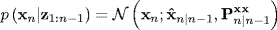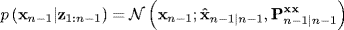# 5.3 The General Bayesian Point Prediction Integrals for Gaussian Densities

Remembering the dynamic equation (5.1), and assuming that vn is a zero-mean Gaussian random variable independent of bothandit follows that

(5.32)Similarly, in the observation equation (5.2), assume that wn is a zero-mean Gaussian random variables independent of both xn and zn, that is

(5.33)Now, from Bayes' law, the prior density can be obtained from

(5.34)makingGaussian, since the product of two Gaussian densities is also Gaussian. Assuming that all density functions are Gaussian, we can identify

(5.35)(5.36)Assuming that vn and wn are zero-mean Gaussian processes, and substituting (5.36) into (5.3) and ...

Get Bayesian Estimation and Tracking: A Practical Guide now with the O’Reilly learning platform.

O’Reilly members experience live online training, plus books, videos, and digital content from nearly 200 publishers.